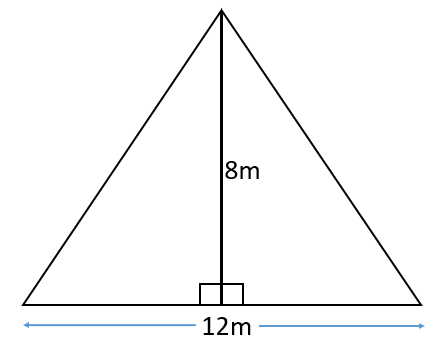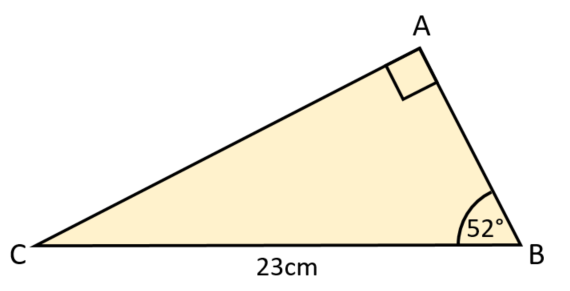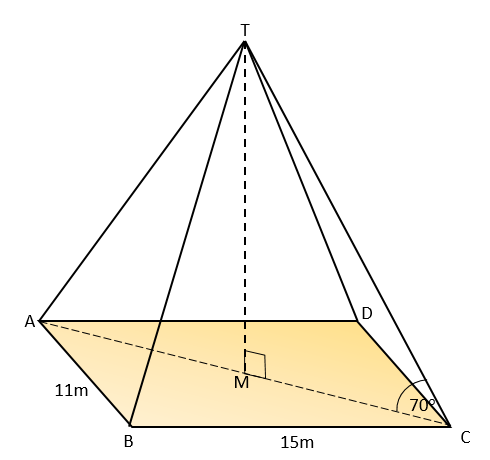# Exam-Style Questions.

## Problems adapted from questions set for previous Mathematics exams.

### 1.

GCSE Higher

An isosceles triangle shaped frame is made from four pieces of metal. The frame has a height of 8 metres and a base of length 12 metres.

The weight of the metal is 2.5kg per metre. Calculate the total weight of the metal in the frame.### 2.

GCSE Higher

ABC is a right-angled triangle as shown in the diagram below. Calculate the length of AB giving your answer correct to three significant figures.### 3.

GCSE Higher

An arborist sights the top of a tree using a clinometer and reads the angle of elevation to be 29o. Her clinometer is 28 metres from the base of the tree and is on a tripod making it 1.5 metres above ground level.

This diagram is not drawn to scale.Calculate the full height of the tree.

### 4.

GCSE Higher

The diagram shows a trapezium where the sides AC and BD are parallel.Calculate the length of side CD

### 5.

GCSE Higher

Four copies of a green right-angled triangle are used to enclose a yellow square.Find the area of the yellow square if the longest side of the green triangle is of length $$a$$ cm and the shortest side is $$b$$ cm.

### 6.

GCSE Higher

The diagram shows a rectangular-based pyramid, TABCD (not drawn to scale).

The horizontal base ABCD has sides of lengths 11m and 15m. The centre of the base of the pyramid is M.

Angle TMC is 90° and angle TCM is 70°

The volume of a pyramid is $$\frac13$$ × area of base × perpendicular height. Calculate the volume of this pyramid.If you would like space on the right of the question to write out the solution try this Thinning Feature. It will collapse the text into the left half of your screen but large diagrams will remain unchanged.

The exam-style questions appearing on this site are based on those set in previous examinations (or sample assessment papers for future examinations) by the major examination boards. The wording, diagrams and figures used in these questions have been changed from the originals so that students can have fresh, relevant problem solving practice even if they have previously worked through the related exam paper.

The solutions to the questions on this website are only available to those who have a Transum Subscription.

Exam-Style Questions Main Page

Search for exam-style questions containing a particular word or phrase:

To search the entire Transum website use the search box in the grey area below.

## Comments:

Do you have any comments about these exam-style questions? It is always useful to receive feedback and helps make this free resource even more useful for those learning Mathematics anywhere in the world. Click here to enter your comments.For All:

©1997-2021 WWW.TRANSUM.ORG